# NCERT Exemplar Solutions for Class 6 Maths Chapter 2 Geometry

NCERT Exemplar Solutions for Class 6 Maths Chapter 2 Geometry, are provided here. It ensures that you get all the necessary information of all concepts included in the chapter. Class 6 is a fundamental level as it forms the base for students for the forthcoming academic years. So, students who aspire to attain good marks in Maths practice NCERT Exemplar Solutions for Class 6 Maths.

Chapter 2 – Geometry is a branch of maths that focuses on the measurement and relationship of lines, angles, surfaces, solids and points. These NCERT Exemplar Class 6 Maths Chapter 2 solutions are available for download in pdf format and provide solutions to all the questions provided in the NCERT Exemplar textbook. In this chapter, students are going to learn some important topics, which are line segment, intersecting line, parallel lines, angle, polygon, triangle, quadrilateral and regular polygon.

## Download the PDF of NCERT Exemplar Solutions for Class 6 Maths Chapter 2 Geometry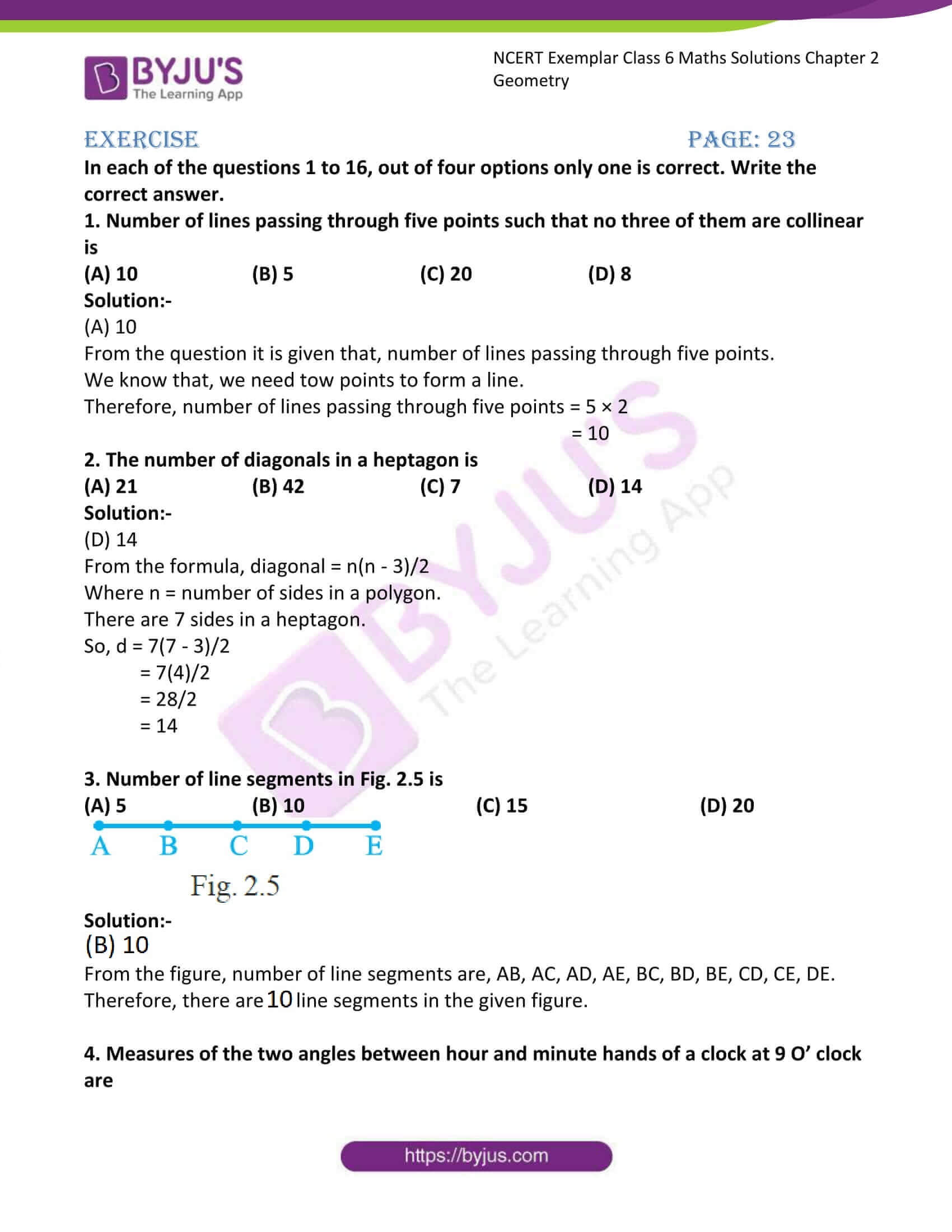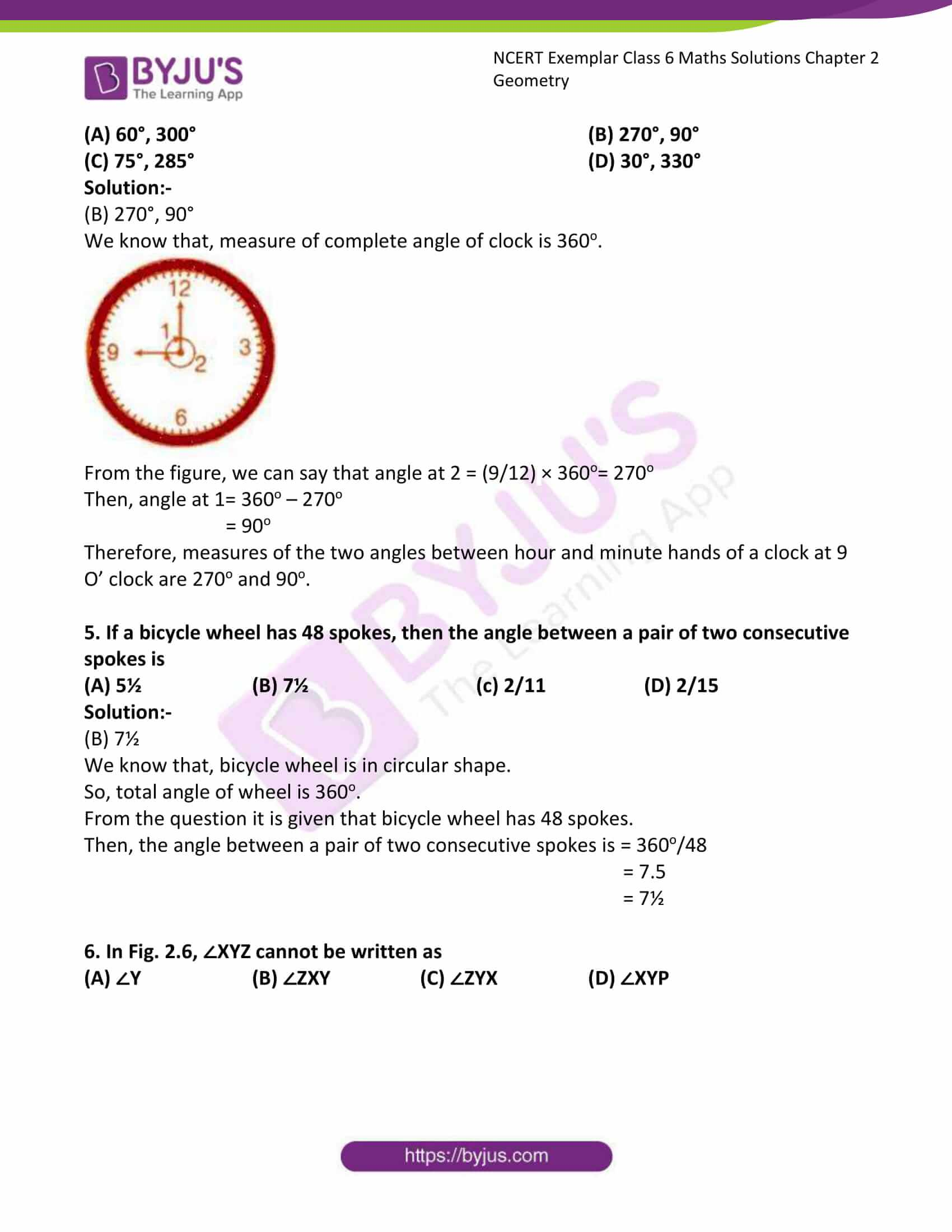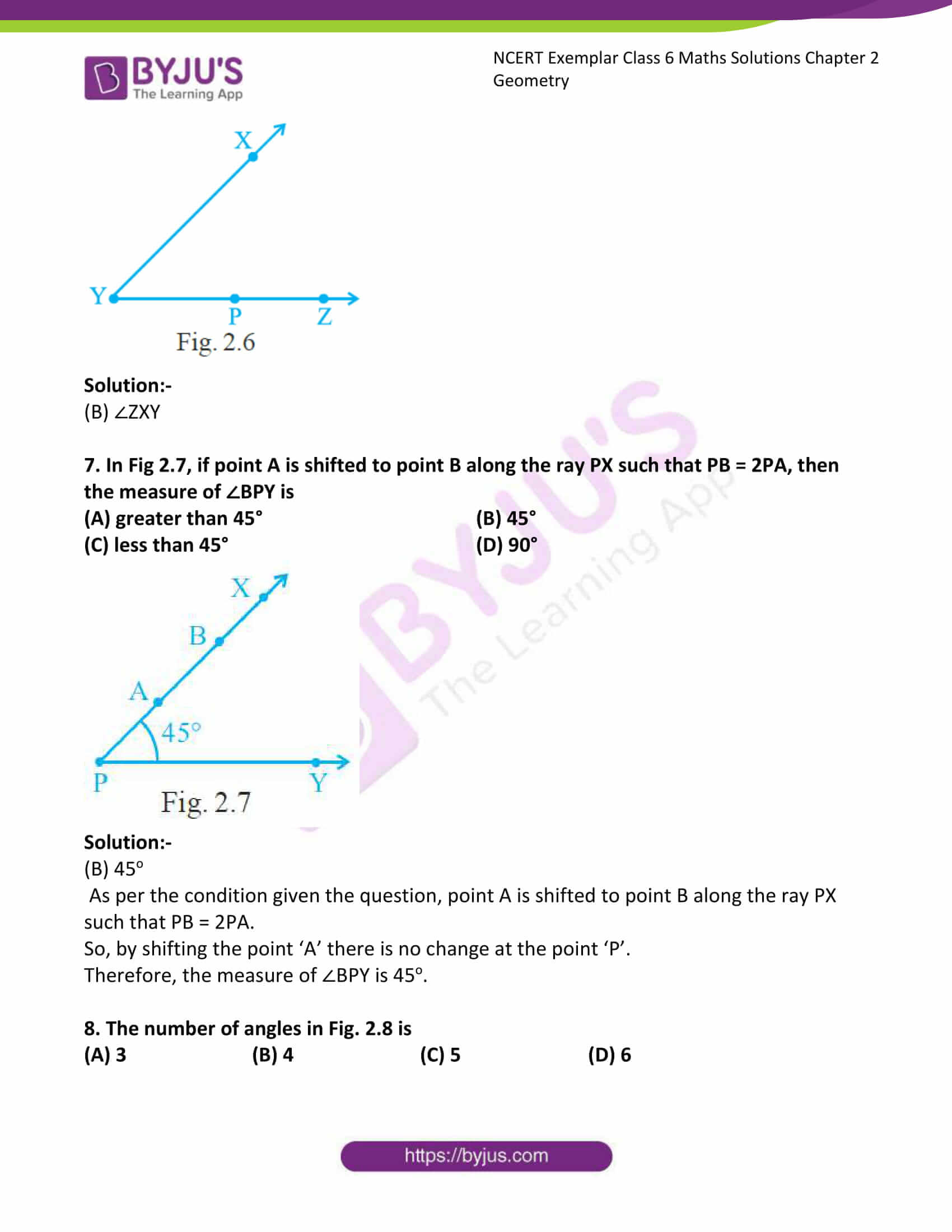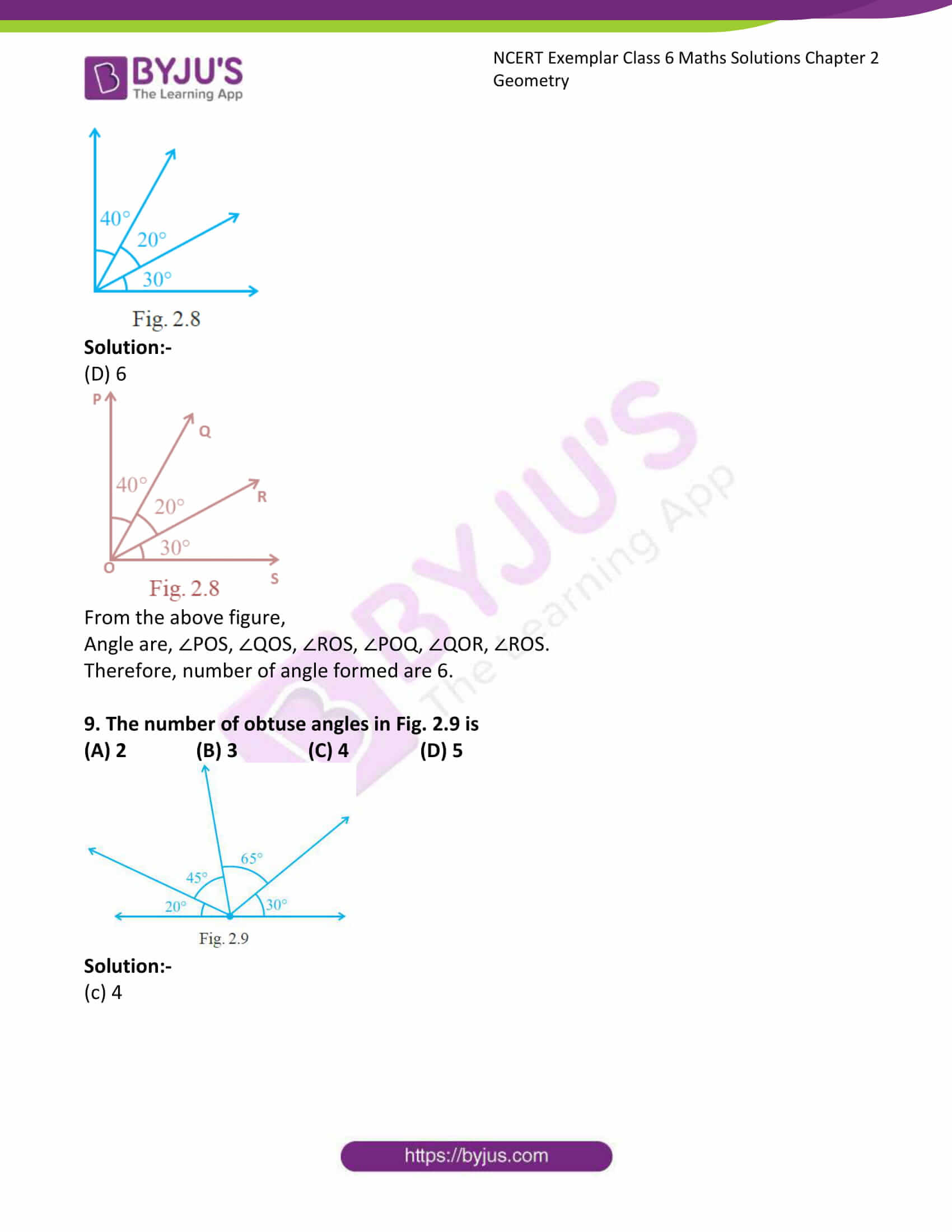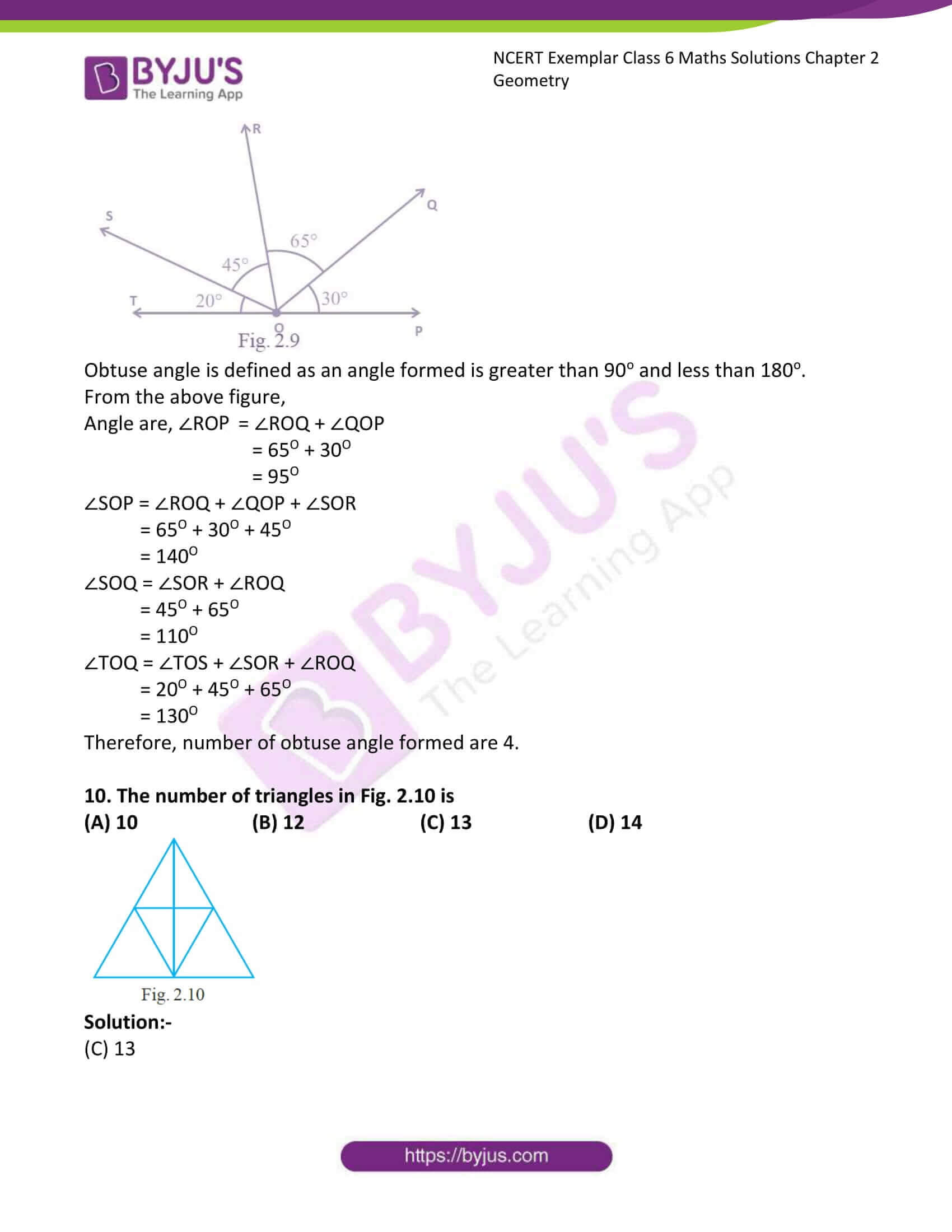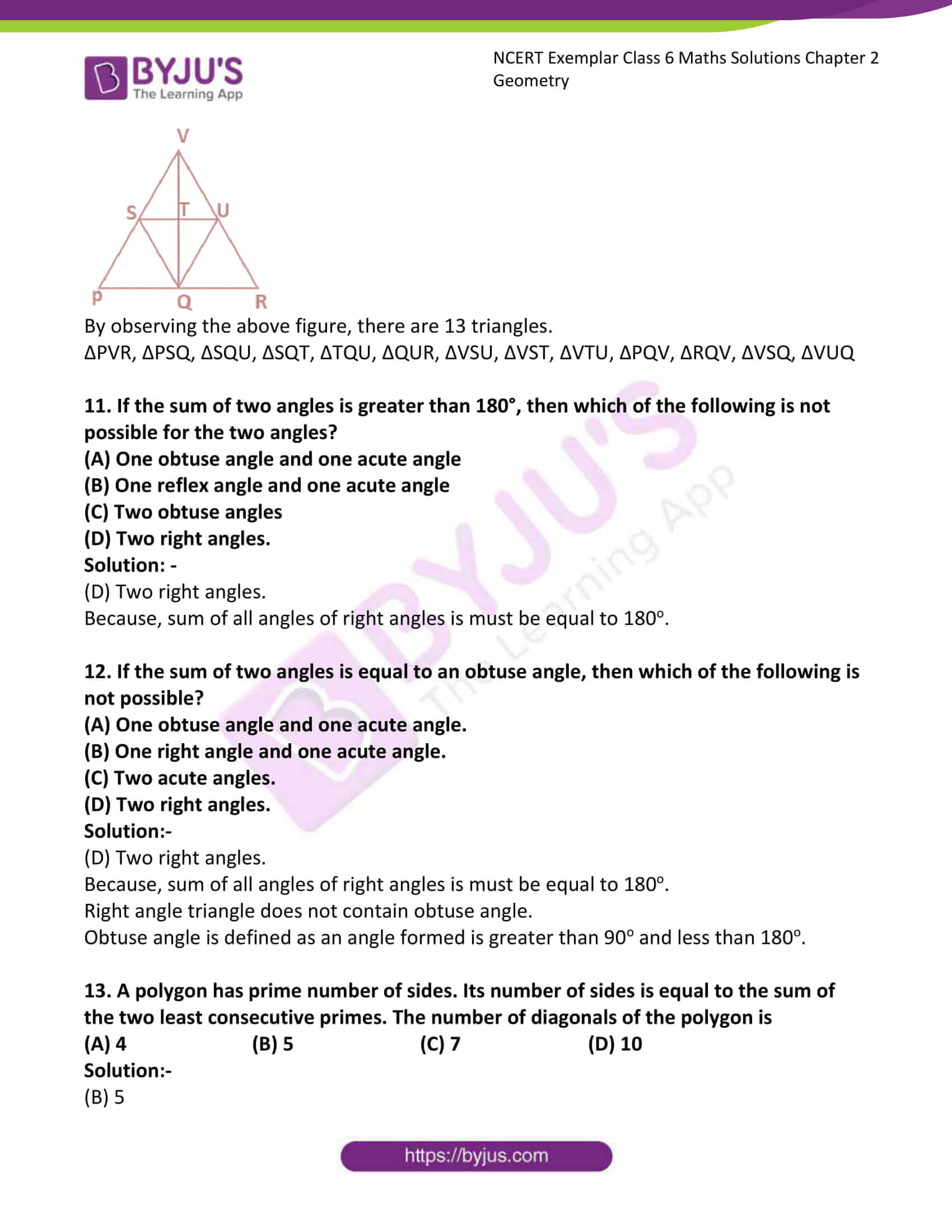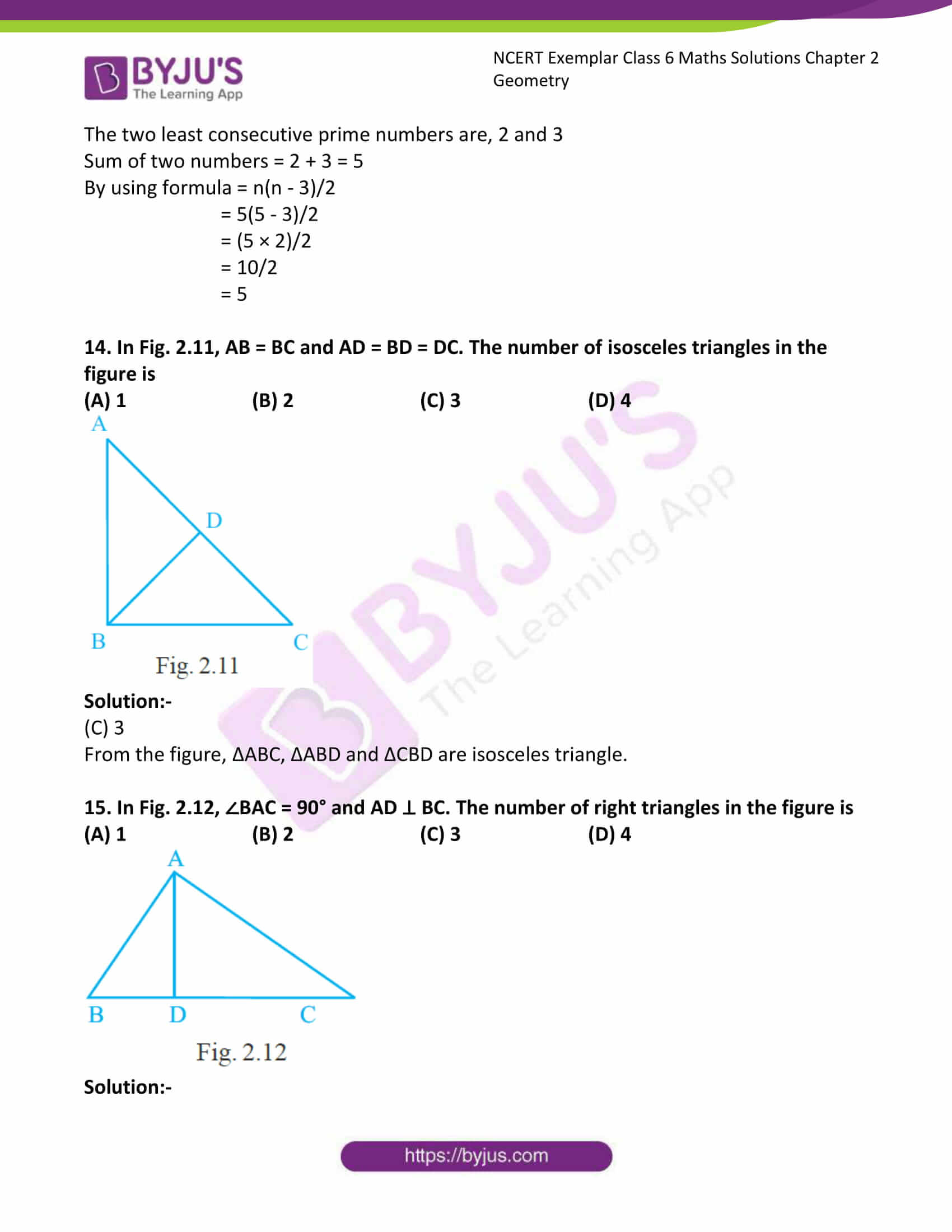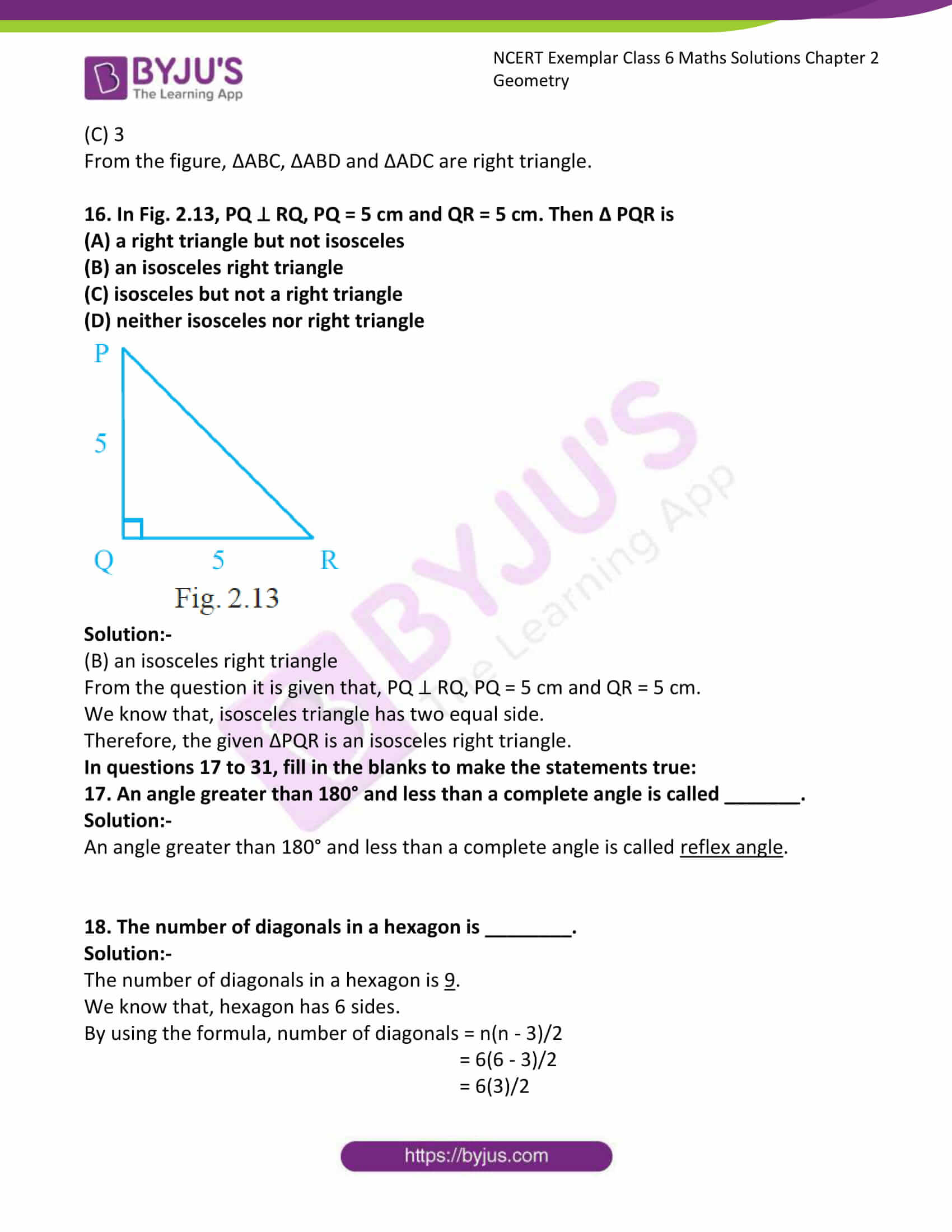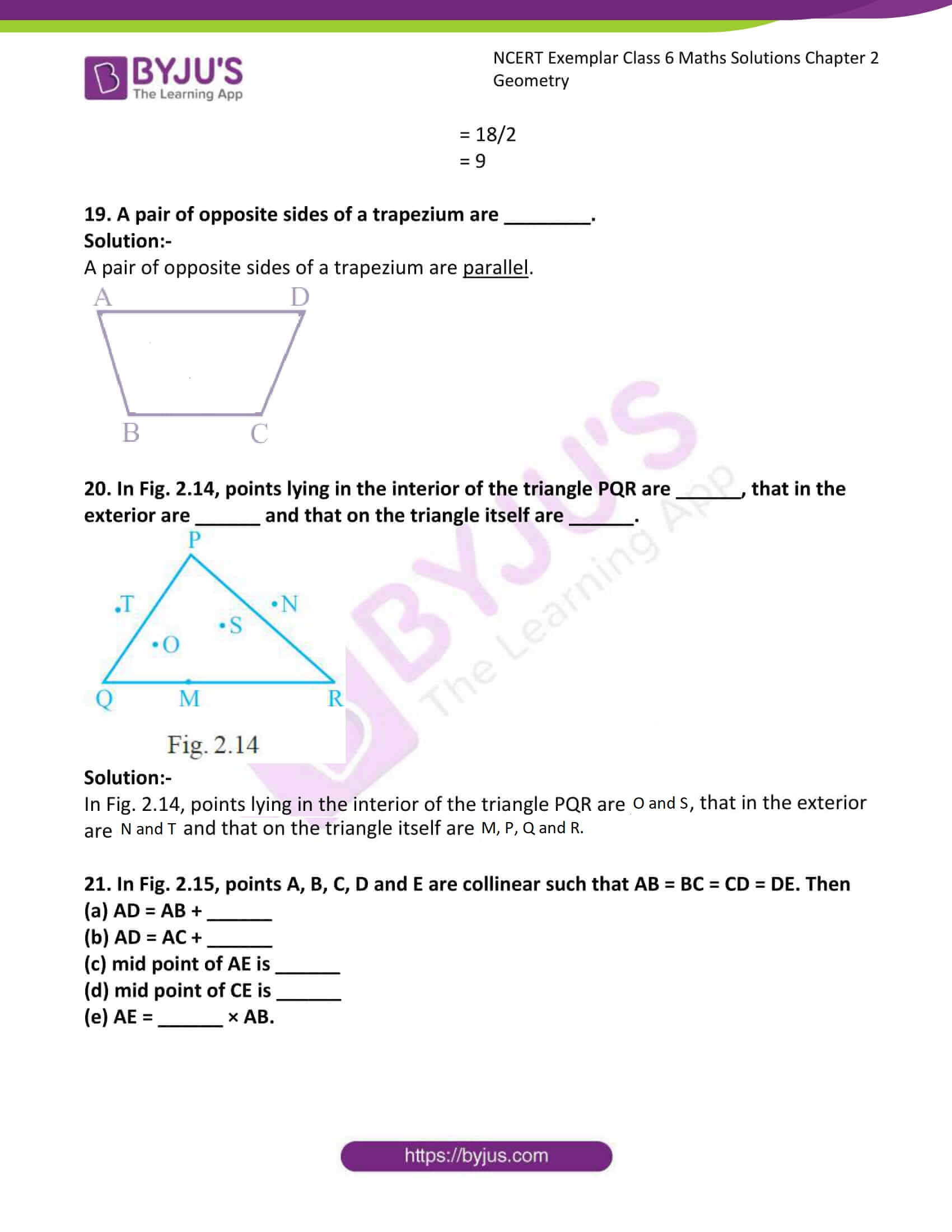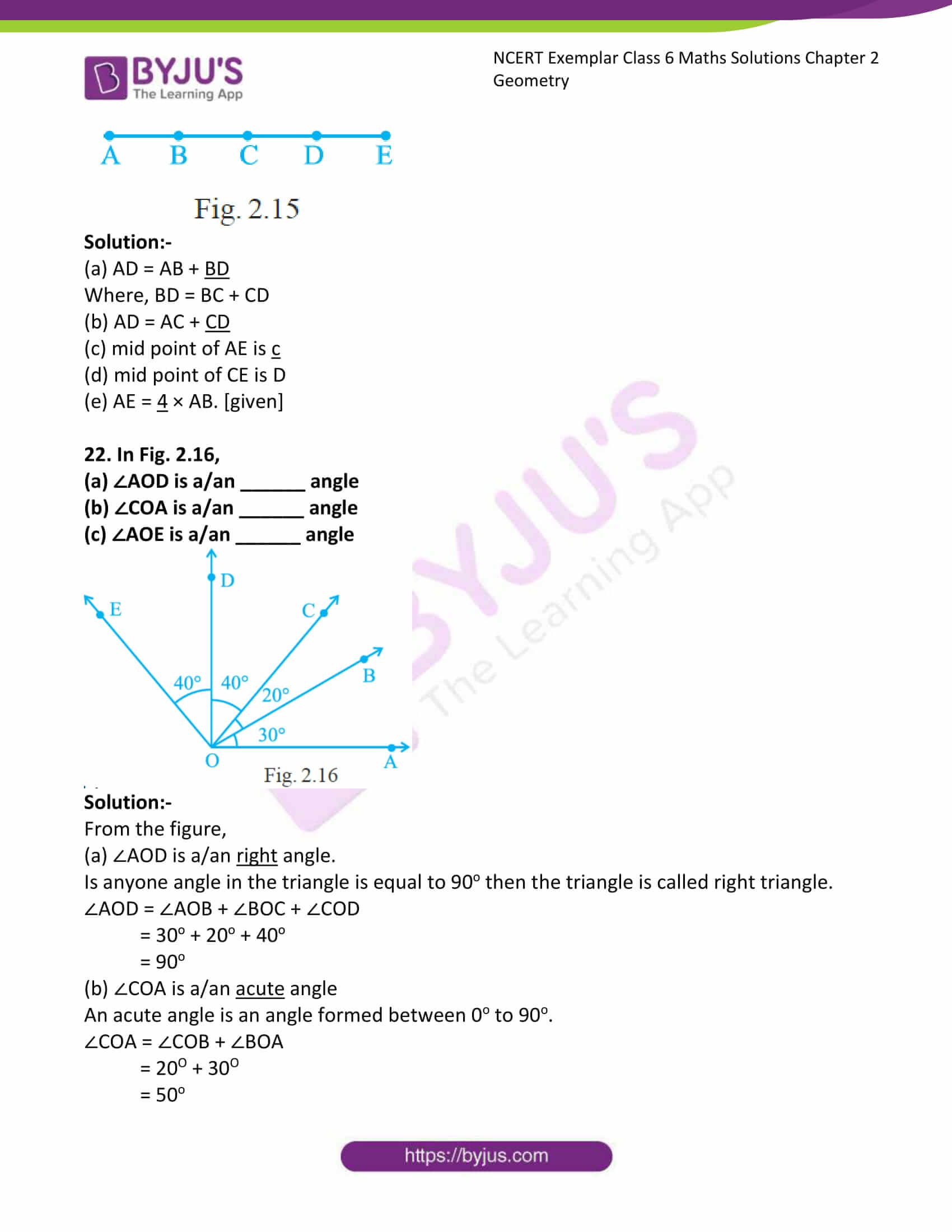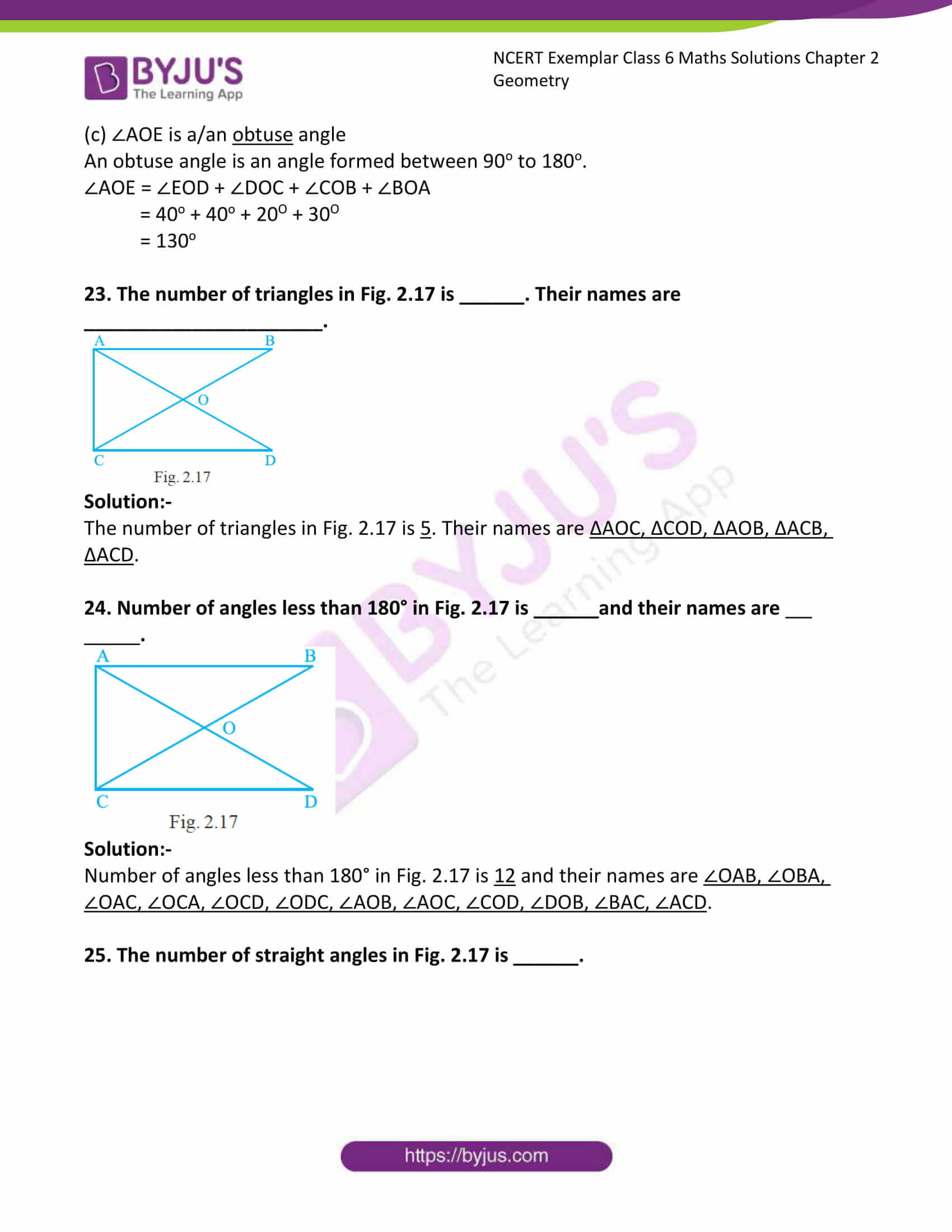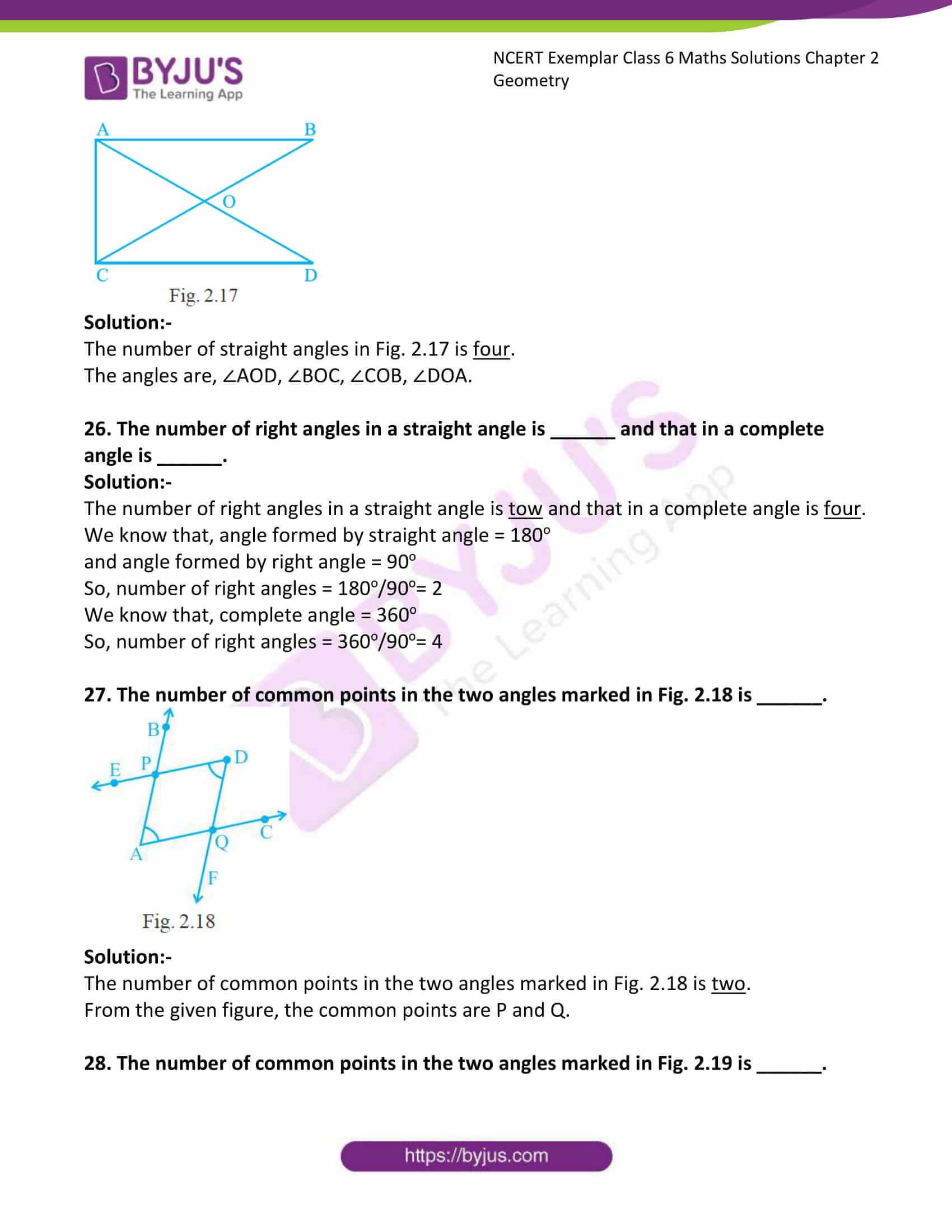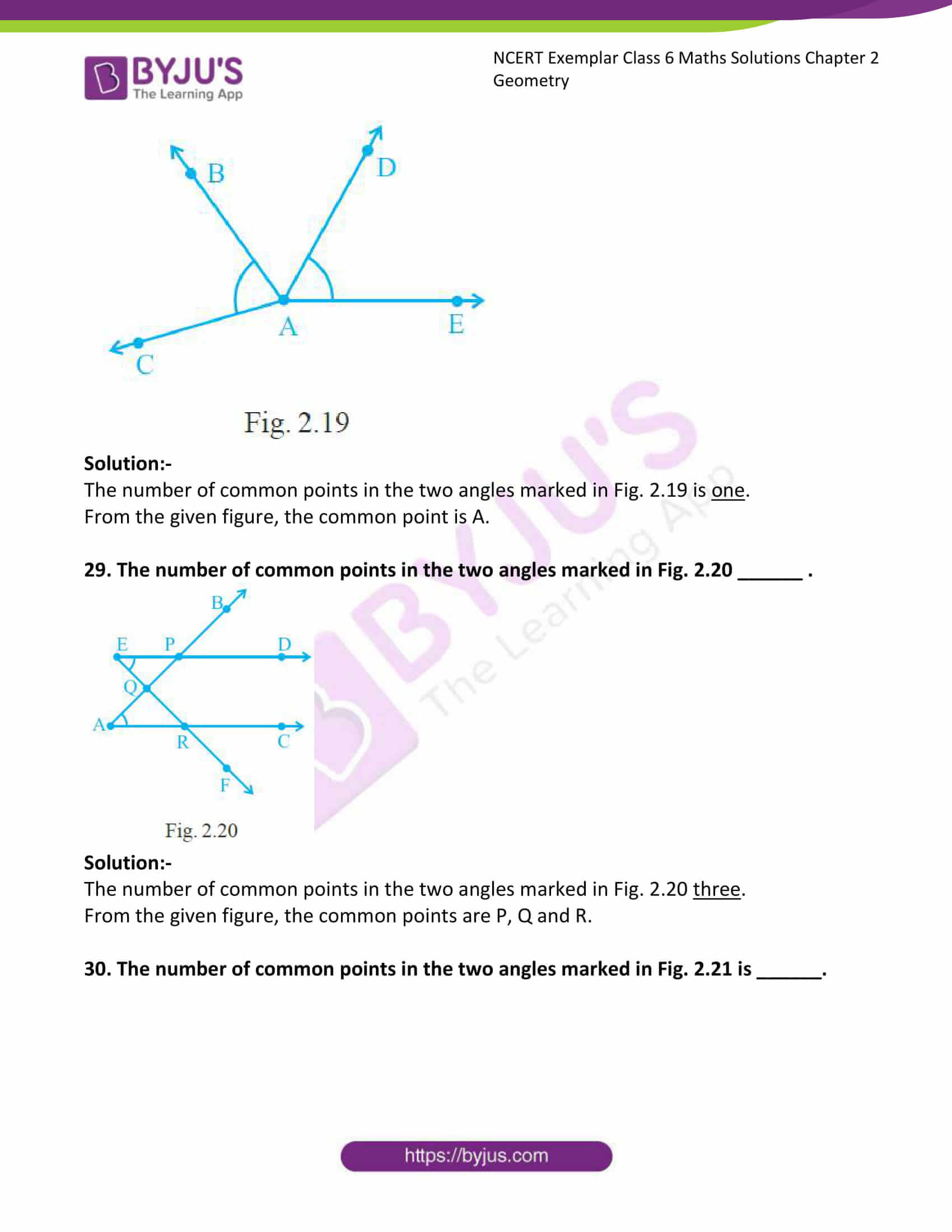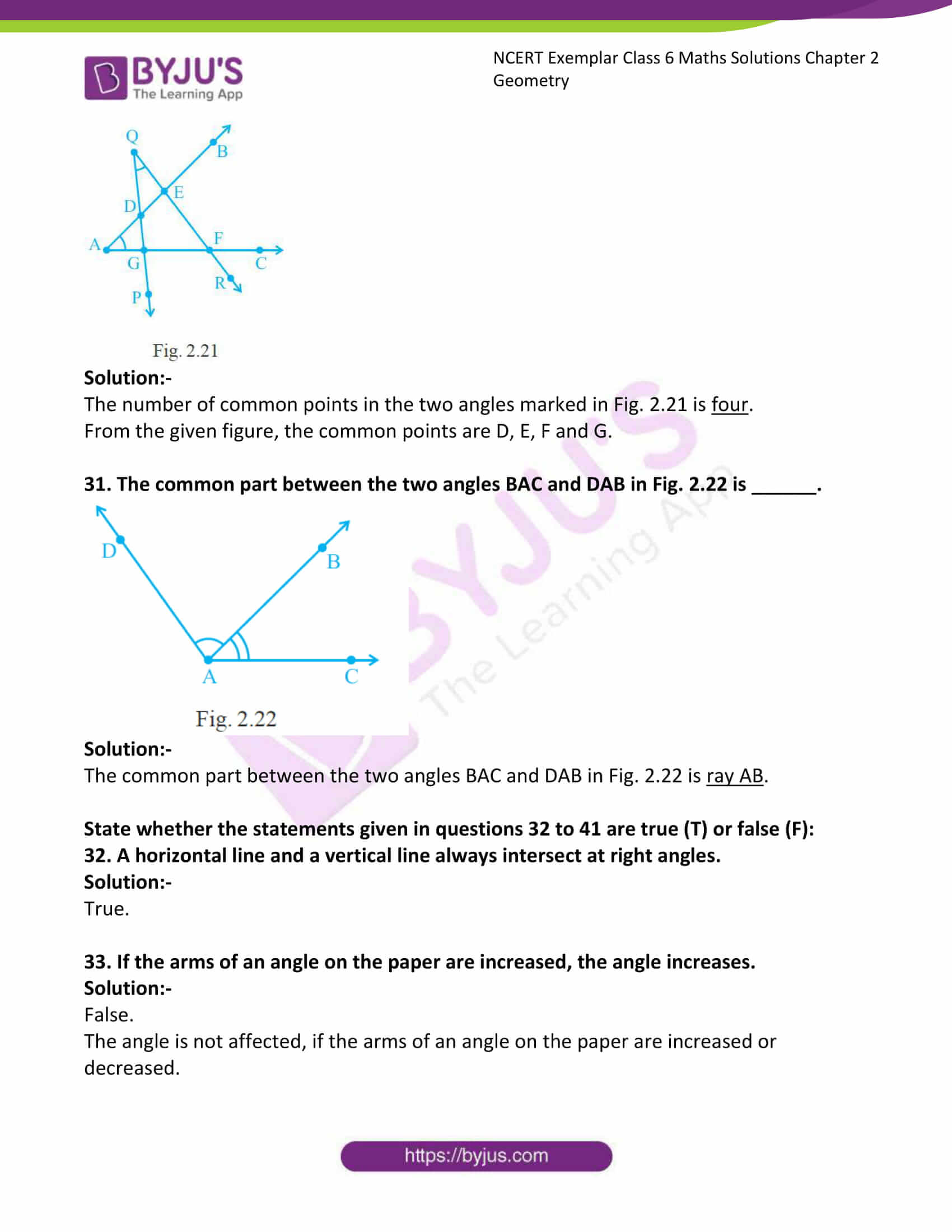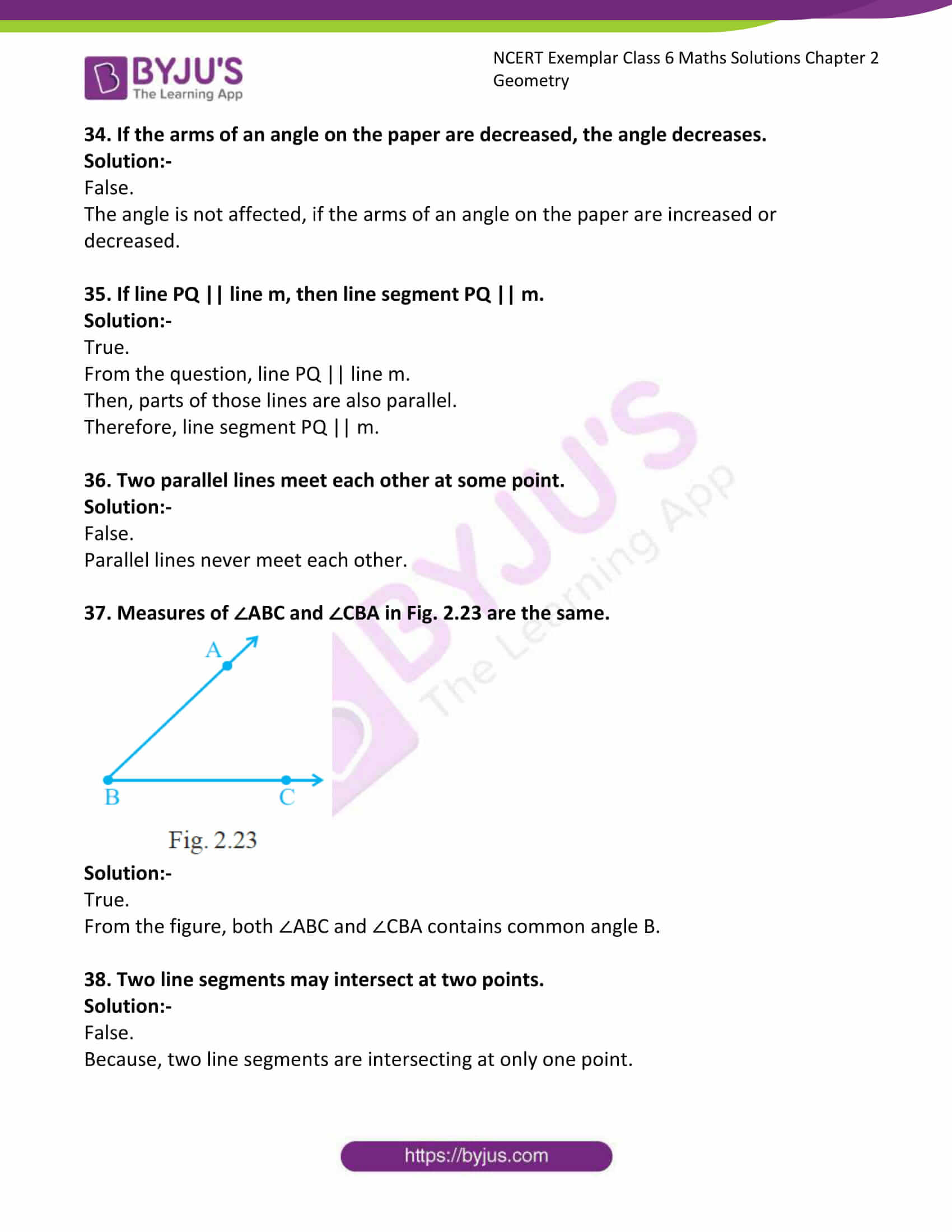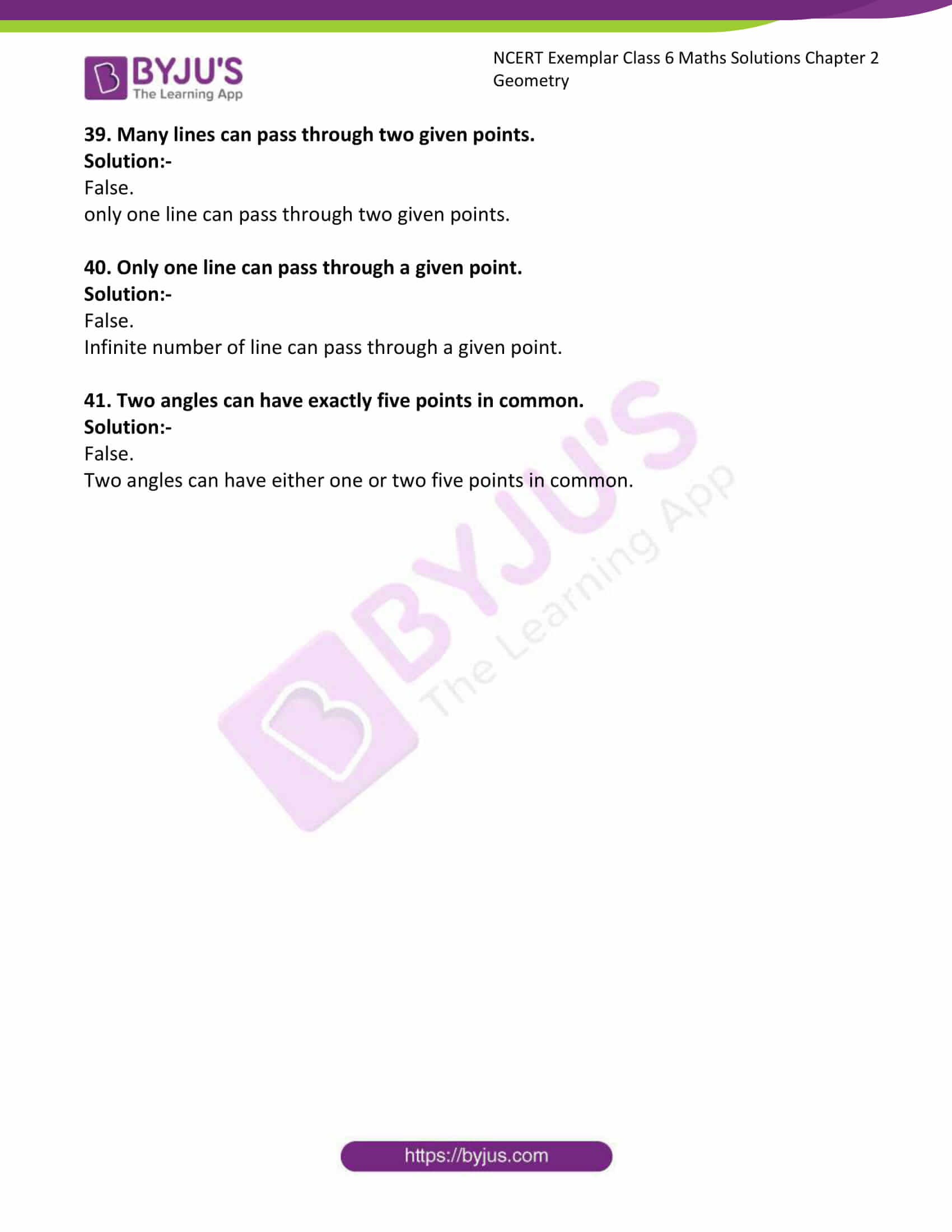### Access Answers to NCERT Exemplar Solutions for Class 6 Maths Chapter 2 Geometry

Exercise Page: 23

In each of the questions 1 to 16, out of four options only one is correct. Write the correct answer.

1. Number of lines passing through five points such that no three of them are collinear is

(A) 10 (B) 5 (C) 20 (D) 8

Solution:-

(A) 10

From the question it is given that, number of lines passing through five points.

We know that, we need tow points to form a line.

Therefore, number of lines passing through five points = 5 × 2

= 10

2. The number of diagonals in a heptagon is

(A) 21 (B) 42 (C) 7 (D) 14

Solution:-

(D) 14

From the formula, diagonal = n(n – 3)/2

Where n = number of sides in a polygon.

There are 7 sides in a heptagon.

So, d = 7(7 – 3)/2

= 7(4)/2

= 28/2

= 14

3. Number of line segments in Fig. 2.5 is

(A) 5 (B) 10 (C) 15 (D) 20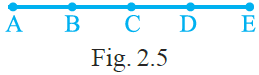Solution:-

(B) 10

From the figure, number of line segments are, AB, AC, AD, AE, BC, BD, BE, CD, CE, DE.

Therefore, there are 10 line segments in the given figure.

4. Measures of the two angles between hour and minute hands of a clock at 9 O’ clock are

(A) 60°, 300° (B) 270°, 90°

(C) 75°, 285° (D) 30°, 330°

Solution:-

(B) 270°, 90°

We know that, measure of complete angle of clock is 360o.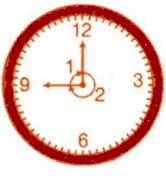From the figure, we can say that angle at 2 = (9/12) × 360o= 270o

Then, angle at 1= 360o – 270o

= 90o

Therefore, measures of the two angles between hour and minute hands of a clock at 9 O’ clock are 270o and 90o.

5. If a bicycle wheel has 48 spokes, then the angle between a pair of two consecutive spokes is

(A) 5½ (B) 7½ (c) 2/11 (D) 2/15

Solution:-

(B) 7½

We know that, bicycle wheel is in circular shape.

So, total angle of wheel is 360o.

From the question it is given that bicycle wheel has 48 spokes.

Then, the angle between a pair of two consecutive spokes is = 360o/48

= 7.5

= 7½

6. In Fig. 2.6, ∠XYZ cannot be written as

(A) ∠Y (B) ∠ZXY (C) ∠ZYX (D) ∠XYP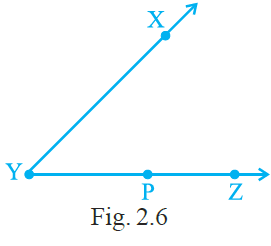Solution:-

(B) ∠ZXY

7. In Fig 2.7, if point A is shifted to point B along the ray PX such that PB = 2PA, then the measure of ∠BPY is

(A) greater than 45° (B) 45°

(C) less than 45° (D) 90°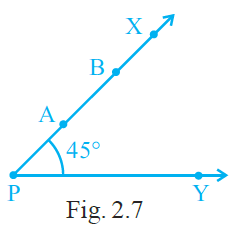Solution:-

(B) 45o

As per the condition given the question, point A is shifted to point B along the ray PX such that PB = 2PA.

So, by shifting the point ‘A’ there is no change at the point ‘P’.

Therefore, the measure of ∠BPY is 45o.

8. The number of angles in Fig. 2.8 is

(A) 3 (B) 4 (C) 5 (D) 6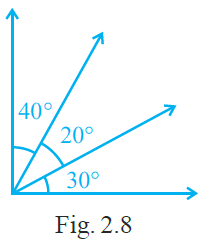Solution:-

(D) 6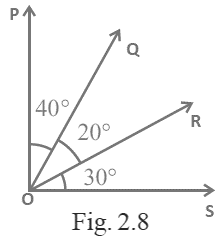From the above figure,

Angle are, ∠POS, ∠QOS, ∠ROS, ∠POQ, ∠QOR, ∠ROS.

Therefore, number of angle formed are 6.

9. The number of obtuse angles in Fig. 2.9 is

(A) 2 (B) 3 (C) 4 (D) 5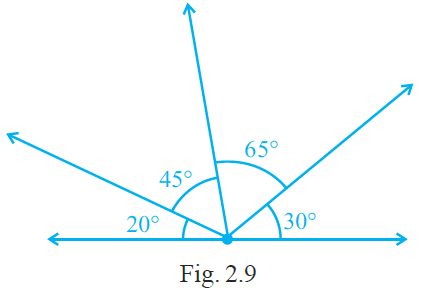Solution:-

(c) 4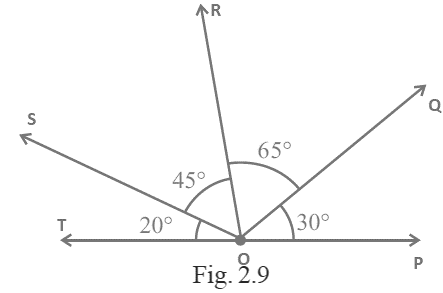Obtuse angle is defined as an angle formed is greater than 90o and less than 180o.

From the above figure,

Angle are, ∠ROP = ∠ROQ + ∠QOP

= 65O + 30O

= 95O

∠SOP = ∠ROQ + ∠QOP + ∠SOR

= 65O + 30O + 45O

= 140O

∠SOQ = ∠SOR + ∠ROQ

= 45O + 65O

= 110O

∠TOQ = ∠TOS + ∠SOR + ∠ROQ

= 20O + 45O + 65O

= 130O

Therefore, number of obtuse angle formed are 4.

10. The number of triangles in Fig. 2.10 is

(A) 10 (B) 12 (C) 13 (D) 14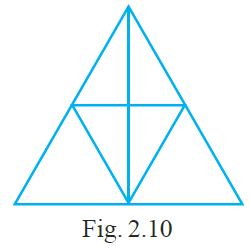Solution:-

(C) 13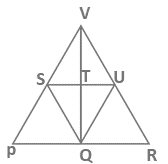By observing the above figure, there are 13 triangles.

ΔPVR, ΔPSQ, ΔSQU, ΔSQT, ΔTQU, ΔQUR, ΔVSU, ΔVST, ΔVTU, ΔPQV, ΔRQV, ΔVSQ, ΔVUQ

11. If the sum of two angles is greater than 180°, then which of the following is not possible for the two angles?

(A) One obtuse angle and one acute angle

(B) One reflex angle and one acute angle

(C) Two obtuse angles

(D) Two right angles.

Solution: –

(D) Two right angles.

Because, sum of all angles of right angles is must be equal to 180o.

12. If the sum of two angles is equal to an obtuse angle, then which of the following is not possible?

(A) One obtuse angle and one acute angle.

(B) One right angle and one acute angle.

(C) Two acute angles.

(D) Two right angles.

Solution:-

(D) Two right angles.

Because, sum of all angles of right angles is must be equal to 180o.

Right angle triangle does not contain obtuse angle.

Obtuse angle is defined as an angle formed is greater than 90o and less than 180o.

13. A polygon has prime number of sides. Its number of sides is equal to the sum of the two least consecutive primes. The number of diagonals of the polygon is

(A) 4 (B) 5 (C) 7 (D) 10

Solution:-

(B) 5

The two least consecutive prime numbers are, 2 and 3

Sum of two numbers = 2 + 3 = 5

By using formula = n(n – 3)/2

= 5(5 – 3)/2

= (5 × 2)/2

= 10/2

= 5

14. In Fig. 2.11, AB = BC and AD = BD = DC. The number of isosceles triangles in the figure is

(A) 1 (B) 2 (C) 3 (D) 4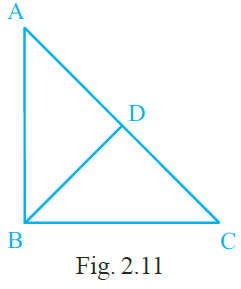Solution:-

(C) 3

From the figure, ΔABC, ΔABD and ΔCBD are isosceles triangle.

15. In Fig. 2.12, ∠BAC = 90° and AD ⊥ BC. The number of right triangles in the figure is

(A) 1 (B) 2 (C) 3 (D) 4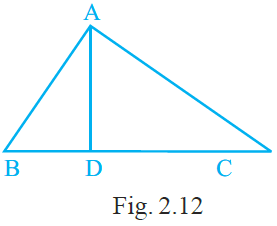Solution:-

(C) 3

From the figure, ΔABC, ΔABD and ΔADC are right triangle.

16. In Fig. 2.13, PQ ⊥ RQ, PQ = 5 cm and QR = 5 cm. Then ∆ PQR is

(A) a right triangle but not isosceles

(B) an isosceles right triangle

(C) isosceles but not a right triangle

(D) neither isosceles nor right triangle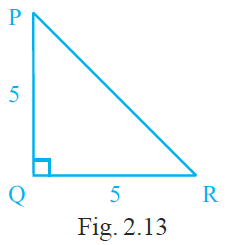Solution:-

(B) an isosceles right triangle

From the question it is given that, PQ ⊥ RQ, PQ = 5 cm and QR = 5 cm.

We know that, isosceles triangle has two equal side.

Therefore, the given ΔPQR is an isosceles right triangle.

In questions 17 to 31, fill in the blanks to make the statements true:

17. An angle greater than 180° and less than a complete angle is called _______.

Solution:-

An angle greater than 180° and less than a complete angle is called reflex angle.

18. The number of diagonals in a hexagon is ________.

Solution:-

The number of diagonals in a hexagon is 9.

We know that, hexagon has 6 sides.

By using the formula, number of diagonals = n(n – 3)/2

= 6(6 – 3)/2

= 6(3)/2

= 18/2

= 9

19. A pair of opposite sides of a trapezium are ________.

Solution:-

A pair of opposite sides of a trapezium are parallel.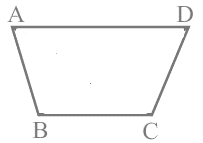20. In Fig. 2.14, points lying in the interior of the triangle PQR are ______, that in the exterior are ______ and that on the triangle itself are ______.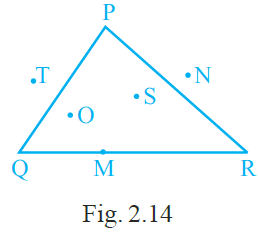Solution:-

In Fig. 2.14, points lying in the interior of the triangle PQR are O and S, that in the exterior are N and T and that on the triangle itself are M, P, Q and R.

21. In Fig. 2.15, points A, B, C, D and E are collinear such that AB = BC = CD = DE. Then

(a) AD = AB + ______

(b) AD = AC + ______

(c) mid point of AE is ______

(d) mid point of CE is ______

(e) AE = ______ × AB.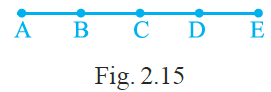Solution:-

(a) AD = AB + BD

Where, BD = BC + CD

(b) AD = AC + CD

(c) mid point of AE is c

(d) mid point of CE is D

(e) AE = 4 × AB. [given]

22. In Fig. 2.16,

(a) ∠AOD is a/an ______ angle

(b) ∠COA is a/an ______ angle

(c) ∠AOE is a/an ______ angle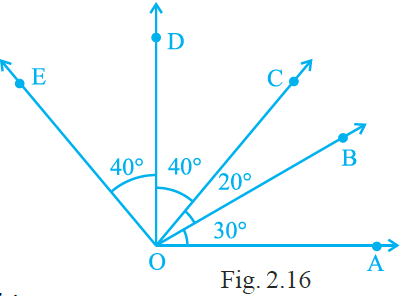Solution:-

From the figure,

(a) ∠AOD is a/an right angle.

Is anyone angle in the triangle is equal to 90o then the triangle is called right triangle.

∠AOD = ∠AOB + ∠BOC + ∠COD

= 30o + 20o + 40o

= 90o

(b) ∠COA is a/an acute angle

An acute angle is an angle formed between 0o to 90o.

∠COA = ∠COB + ∠BOA

= 20O + 30O

= 50o

(c) ∠AOE is a/an obtuse angle

An obtuse angle is an angle formed between 90o to 180o.

∠AOE = ∠EOD + ∠DOC + ∠COB + ∠BOA

= 40o + 40o + 20O + 30O

= 130o

23. The number of triangles in Fig. 2.17 is ______. Their names are ______________________.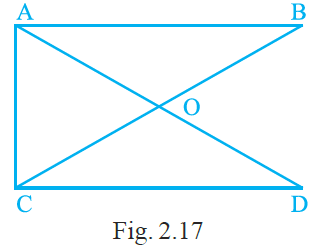Solution:-

The number of triangles in Fig. 2.17 is 5. Their names are ΔAOC, ΔCOD, ΔAOB, ΔACB, ΔACD.

24. Number of angles less than 180° in Fig. 2.17 is ______and their names are .Solution:-

Number of angles less than 180° in Fig. 2.17 is 12 and their names are ∠OAB, ∠OBA, ∠OAC, ∠OCA, ∠OCD, ∠ODC, ∠AOB, ∠AOC, ∠COD, ∠DOB, ∠BAC, ∠ACD.

25. The number of straight angles in Fig. 2.17 is ______.Solution:-

The number of straight angles in Fig. 2.17 is four.

The angles are, ∠AOD, ∠BOC, ∠COB, ∠DOA.

26. The number of right angles in a straight angle is ______ and that in a complete angle is ______.

Solution:-

The number of right angles in a straight angle is tow and that in a complete angle is four.

We know that, angle formed by straight angle = 180o

and angle formed by right angle = 90o

So, number of right angles = 180o/90o= 2

We know that, complete angle = 360o

So, number of right angles = 360o/90o= 4

27. The number of common points in the two angles marked in Fig. 2.18 is ______.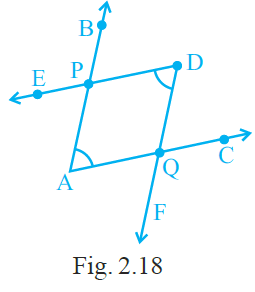Solution:-

The number of common points in the two angles marked in Fig. 2.18 is two.

From the given figure, the common points are P and Q.

28. The number of common points in the two angles marked in Fig. 2.19 is ______.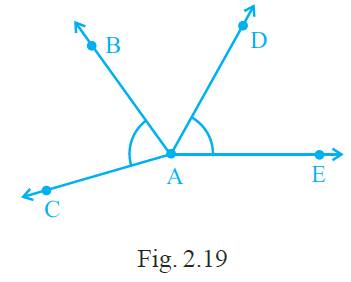Solution:-

The number of common points in the two angles marked in Fig. 2.19 is one.

From the given figure, the common point is A.

29. The number of common points in the two angles marked in Fig. 2.20 ______ .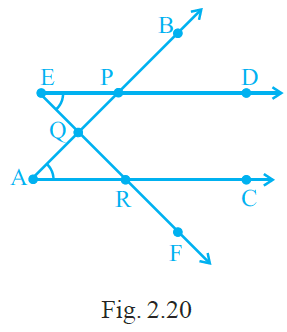Solution:-

The number of common points in the two angles marked in Fig. 2.20 three.

From the given figure, the common points are P, Q and R.

30. The number of common points in the two angles marked in Fig. 2.21 is ______.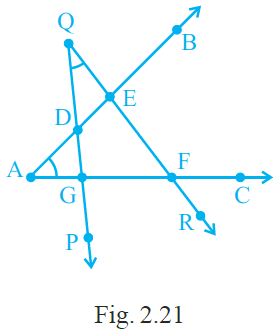Solution:-

The number of common points in the two angles marked in Fig. 2.21 is four.

From the given figure, the common points are D, E, F and G.

31. The common part between the two angles BAC and DAB in Fig. 2.22 is ______.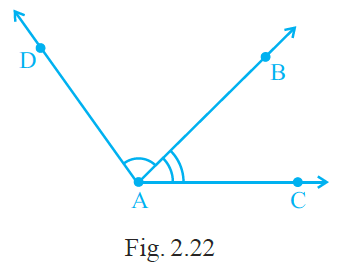Solution:-

The common part between the two angles BAC and DAB in Fig. 2.22 is ray AB.

State whether the statements given in questions 32 to 41 are true (T) or false (F):

32. A horizontal line and a vertical line always intersect at right angles.

Solution:-

True.

33. If the arms of an angle on the paper are increased, the angle increases.

Solution:-

False.

The angle is not affected, if the arms of an angle on the paper are increased or decreased.

34. If the arms of an angle on the paper are decreased, the angle decreases.

Solution:-

False.

The angle is not affected, if the arms of an angle on the paper are increased or decreased.

35. If line PQ || line m, then line segment PQ || m.

Solution:-

True.

From the question, line PQ || line m.

Then, parts of those lines are also parallel.

Therefore, line segment PQ || m.

36. Two parallel lines meet each other at some point.

Solution:-

False.

Parallel lines never meet each other.

37. Measures of ∠ABC and ∠CBA in Fig. 2.23 are the same.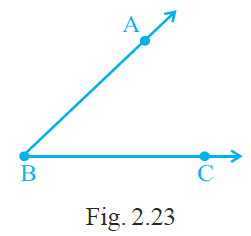Solution:-

True.

From the figure, both ∠ABC and ∠CBA contains common angle B.

38. Two line segments may intersect at two points.

Solution:-

False.

Because, two line segments are intersecting at only one point.

39. Many lines can pass through two given points.

Solution:-

False.

only one line can pass through two given points.

40. Only one line can pass through a given point.

Solution:-

False.

Infinite number of line can pass through a given point.

41. Two angles can have exactly five points in common.

Solution:-

False.

Two angles can have either one or two five points in common.